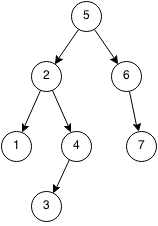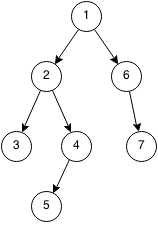## Introduction

Prerequisites: Recursion, Stack

A depth first search (DFS) is a search that goes as far as possible before backtracking. The search requires a stack but DFS is usually implemented with recursion which uses the system stack. So most of the time you do not need an explicit stack.

1. Push the root into the stack.
2. Pop the first element from the stack and push all of its non-visited neighbours to the stack.
3. Repeat until the stack is empty.## Implementation

Most of the time, DFS is implemented using recursion and it is very short and simple to code.

```public class Tree {
int value;
Tree left;
Tree right;
}
```

### Binary Tree TraversalImplementation for outputting a binary tree in order from left to right using DFS:

```public static void DFS(Tree cur) {
if (cur == null) {
return;
}
DFS(cur.left);
System.out.println(cur.value);
DFS(cur.right);
}
```

### Binary Tree PreorderImplementation for outputting a binary tree in DFS pre order:

```public static void DFS(Tree cur) {
if (cur == null) {
return;
}
System.out.println(cur.value);
DFS(cur.left);
DFS(cur.right);
}
```

### Binary Tree PostorderImplementation for outputting a binary tree in DFS postorder:

```public static void DFS(Tree cur) {
if (cur == null) {
return;
}
DFS(cur.left);
DFS(cur.right);
System.out.println(cur.value);
}
```

### Graph Traversal

This is a DFS implementation for traversing a bidirectional graph with positive weights:

```public static void DFS_graph(int[][] adjMatrix, int cur, boolean[] visited) {
if (visited[cur]) {
return;
}
visited[cur] = true;
System.out.println(cur);
for (int i = 0; i < adjMatrix.length; i++) {